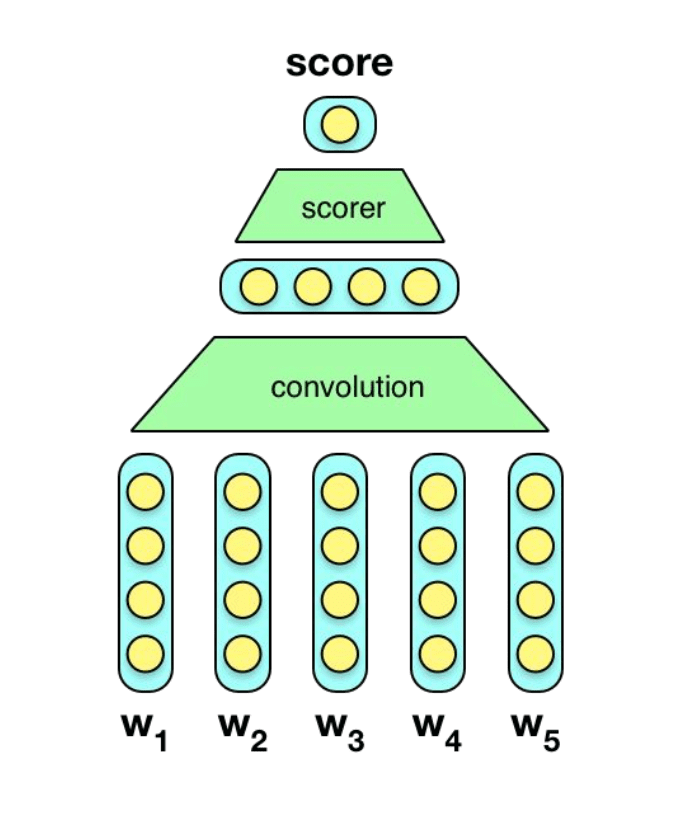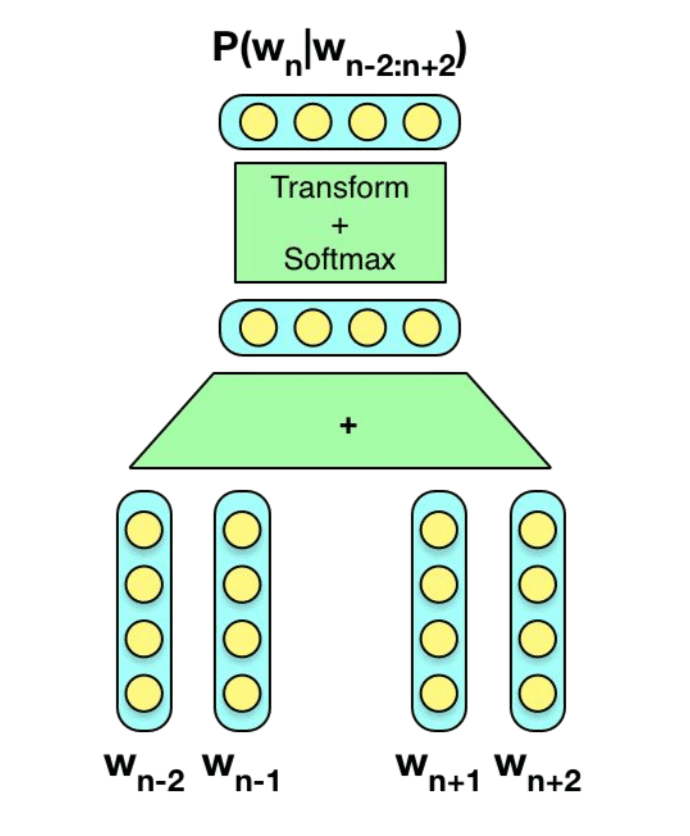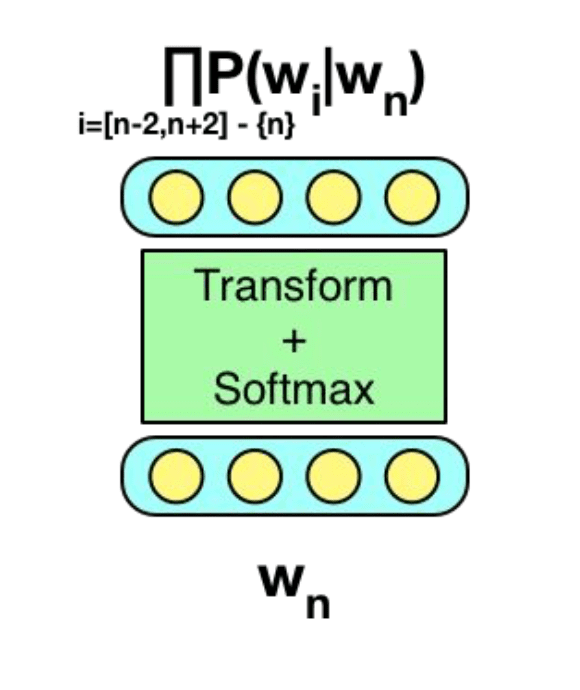# NIUHE

## Word Level Semantics

### Count-based methods

• Define a basis vocabulary $C$ of context words
• Define a word window size $w$
• Count the basis vocabulary words occurring $w$ words to the left or right of each instance of a target word in the corpus.
• Form a vector representation of the target word based on these counts.

Example:

• ... and the cute kitten purred and then ...
• ... the cute furry cat purred and miaowed ...
• ... that the small kitten miaowed and she ...
• ... the loud furry dog ran and bit ...

Example basis vocabulary: {bit, cute, furry, loud, miaowed, purred, ran, small}.

• kitten context words: {cute, purred, small, miaowed}.
• cat context words: {cute, furry, miaowed}.
• dog context words: {loud, furry, ran, bit}.

Therefore

• $kitten = [0, 1, 0, 0, 1, 1, 0, 1]^T$
• $cat = [0, 1, 1, 0, 1, 0, 0, 0]^T$
• $dog = [1, 0, 1, 1, 0, 0, 1, 0]^T$

### Neural Embedding Models

• Learning count based vetors produces an embedding matrix $E$ in $R^{|vocab|*|context|}$.• Rows are word vectors = one hot vector

• $cat = onehot^T_{cat}E$

General idea behind embedding learning:

1. Collect instances $t_i \in inst(t)$ of a word $t$ of vocab $V$
2. For each instance, collect its context words $c(t_i)$ (e.g. k-word window)
3. Define some score function $socre(t_i, c(t_i); \theta, E)$ with upper bound on output
4. Define a loss
5. Estimate
6. Use the estimated $E$ as your embedding matrix

#### C&W#### CBoWword2vec详解：http://blog.csdn.net/itplus/article/details/37969979

#### Skip-gramNeural network parameters are updated using gradients on loss $L(x, y, \theta)$ $\theta_{t+1} = update(\theta_t, \triangledown_\theta L(x, y, \theta_t))$ If $E \in \theta$ then this update can modify $E$ : $E_{t+1} = update(E_t, \triangledown_E L(x, y, \theta_t))$ General intuition: learn to classify/predict/generate based on features, but also the features themselves.